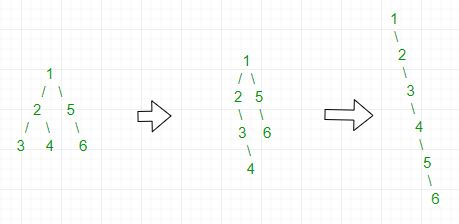# Flatten a binary tree into linked list

Given a binary tree, flatten it into linked list in-place. Usage of auxiliary data structure is not allowed. After flattening, left of each node should point to NULL and right should contain next node in preorder.
Examples:

```Input :
1
/   \
2     5
/ \     \
3   4     6

Output :
1
\
2
\
3
\
4
\
5
\
6

Input :
1
/ \
3   4
/
2
\
5
Output :
1
\
3
\
4
\
2
\
5

```

Simple Approach: A simple solution is to use Level Order Traversal using Queue. In level order traversal, keep track of previous node. Make current node as right child of previous and left of previous node as NULL. This solution requires queue, but question asks to solve without additional data structure.
Efficient Without Additional Data Structure Recursively look for the node with no grandchildren and both left and right child in the left sub-tree. Then store node->right in temp and make node->right=node->left. Insert temp in first node NULL on right of node by node=node->right. Repeat until it is converted to linked list.
For Example,## C++

 `/* Program to flatten a given Binary ` `   ``Tree into linked list */` `#include ` `using` `namespace` `std;`   `struct` `Node {` `    ``int` `key;` `    ``Node *left, *right;` `};`   `/* utility that allocates a new Node ` `   ``with the given key  */` `Node* newNode(``int` `key)` `{` `    ``Node* node = ``new` `Node;` `    ``node->key = key;` `    ``node->left = node->right = NULL;` `    ``return` `(node);` `}`   `// Function to convert binary tree into` `// linked list by altering the right node` `// and making left node point to NULL` `void` `flatten(``struct` `Node* root)` `{` `    ``// base condition- return if root is NULL` `    ``// or if it is a leaf node` `    ``if` `(root == NULL || root->left == NULL &&` `                        ``root->right == NULL) {` `        ``return``;` `    ``}`   `    ``// if root->left exists then we have ` `    ``// to make it root->right` `    ``if` `(root->left != NULL) {`   `        ``// move left recursively` `        ``flatten(root->left);` `   `  `        ``// store the node root->right` `        ``struct` `Node* tmpRight = root->right;` `        ``root->right = root->left;` `        ``root->left = NULL;`   `        ``// find the position to insert` `        ``// the stored value   ` `        ``struct` `Node* t = root->right;` `        ``while` `(t->right != NULL) {` `            ``t = t->right;` `        ``}`   `        ``// insert the stored value` `        ``t->right = tmpRight;` `    ``}`   `    ``// now call the same function` `    ``// for root->right` `    ``flatten(root->right);` `}`   `// To find the inorder traversal` `void` `inorder(``struct` `Node* root)` `{` `    ``// base condition` `    ``if` `(root == NULL)` `        ``return``;` `    ``inorder(root->left);` `    ``cout << root->key << ``" "``;` `    ``inorder(root->right);` `}`   `/* Driver program to test above functions*/` `int` `main()` `{` `    ``/*    1` `        ``/   \` `       ``2     5` `      ``/ \     \` `     ``3   4     6 */` `    ``Node* root = newNode(1);` `    ``root->left = newNode(2);` `    ``root->right = newNode(5);` `    ``root->left->left = newNode(3);` `    ``root->left->right = newNode(4);` `    ``root->right->right = newNode(6);`   `    ``flatten(root);`   `    ``cout << ``"The Inorder traversal after "` `            ``"flattening binary tree "``;` `    ``inorder(root);` `    ``return` `0;` `}`

## Java

 `// Java program to flatten a given ` `// Binary Tree into linked list`   `// A binary tree node` `class` `Node ` `{` `    ``int` `data;` `    ``Node left, right;`   `    ``Node(``int` `key)` `    ``{` `        ``data = key;` `        ``left = right = ``null``;` `    ``}` `}`   `class` `BinaryTree{` `    `  `Node root;`   `// Function to convert binary tree into` `// linked list by altering the right node` `// and making left node NULL` `public` `void` `flatten(Node node) ` `{` `    `  `    ``// Base case - return if root is NULL` `    ``if` `(node == ``null``)` `        ``return``;`   `    ``// Or if it is a leaf node` `    ``if` `(node.left == ``null` `&&` `       ``node.right == ``null``)` `        ``return``;`   `    ``// If root.left children exists then we have` `    ``// to make it node.right (where node is root)` `    ``if` `(node.left != ``null``)` `    ``{` `        `  `        ``// Move left recursively` `        ``flatten(node.left);`   `        ``// Store the node.right in ` `        ``// Node named tempNode` `        ``Node tempNode = node.right;` `        ``node.right = node.left;` `        ``node.left = ``null``;`   `        ``// Find the position to insert` `        ``// the stored value` `        ``Node curr = node.right;` `        ``while` `(curr.right != ``null``) ` `        ``{` `            ``curr = curr.right;` `        ``}`   `        ``// Insert the stored value` `        ``curr.right = tempNode;` `    ``}`   `    ``// Now call the same function` `    ``// for node.right` `    ``flatten(node.right);`   `}`   `// Function for Inorder traversal` `public` `void` `inOrder(Node node)` `{` `    `  `    ``// Base Condition` `    ``if` `(node == ``null``)` `        ``return``;`   `    ``inOrder(node.left);` `    ``System.out.print(node.data + ``" "``);` `    ``inOrder(node.right);` `}`   `// Driver code` `public` `static` `void` `main(String[] args)` `{` `    ``BinaryTree tree = ``new` `BinaryTree();`   `    ``/*    1` `        ``/   \` `       ``2     5` `      ``/ \     \` `     ``3   4     6 */`   `    ``tree.root = ``new` `Node(``1``);` `    ``tree.root.left = ``new` `Node(``2``);` `    ``tree.root.right = ``new` `Node(``5``);` `    ``tree.root.left.left = ``new` `Node(``3``);` `    ``tree.root.left.right = ``new` `Node(``4``);` `    ``tree.root.right.right = ``new` `Node(``6``);`   `    ``System.out.println(``"The Inorder traversal after "` `+` `                       ``"flattening binary tree "``);` `                       `  `    ``tree.flatten(tree.root);` `    ``tree.inOrder(tree.root);` `}` `}`   `// This code is contributed by Yash Singhal`

Output:

```The Inorder traversal after flattening
binary tree 1 2 3 4 5 6

```

Attention reader! Don’t stop learning now. Get hold of all the important DSA concepts with the DSA Self Paced Course at a student-friendly price and become industry ready.

My Personal Notes arrow_drop_upStudent at Netaji Subhas Institute of Technology

If you like GeeksforGeeks and would like to contribute, you can also write an article using contribute.geeksforgeeks.org or mail your article to contribute@geeksforgeeks.org. See your article appearing on the GeeksforGeeks main page and help other Geeks.

Please Improve this article if you find anything incorrect by clicking on the "Improve Article" button below.

Improved By : Spider_man, ysinghal555